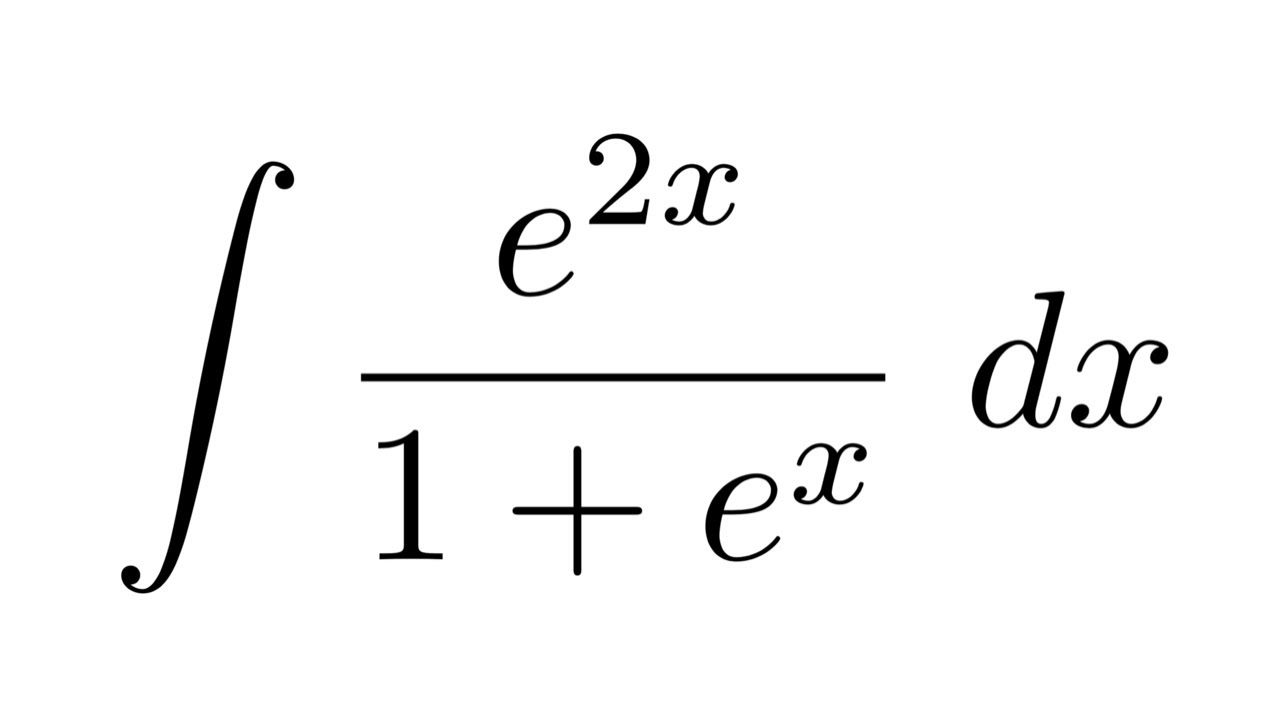June 6, 2023

# Integral of e to the 2xLet’s review some information about e2x before attempting to determine the integral of e to the 2x. It is an exponential function since its exponent contains a variable and its base, “e,” which is a constant (sometimes referred to as Euler’s number). It can alternatively be written as e2x. To get the integral of e to the 2x, we use methods for integrating exponential functions.

Also Read: –What Are The 5 Layers Of Soil

Let’s use various techniques to determine the integral of e to the 2x and use this information to solve a few cases.

## What is the Integral of e to the 2x?

The integral of e to the 2x is e2x/2 + C. This is mathematically written as ∫ e2x dx = e2x/2 + C. Here,

• ‘∫’ is the symbol of integration.
• e2x that is next to dx is the integrand.
• C is the integration constant which is written along with the indefinite integral value of any function.

### Common Illusion Regarding Integral of e to the 2x

Do not assume that e2x dx is e2x + C since ex dx = ex + C. The real integral value must always be divided by the x-coefficient. Because x has a coefficient of 2, e2x dx = e2x/2 + C.

Let’s use several techniques to demonstrate that the integral of e to the 2x is e2x/2 + C and then use differentiation to confirm the outcome.

### Integral of e to the 2x by Differentiation

We are aware that differentiation and integration operate in the opposite directions from one another. Additionally, we are aware that the integral of a derivative can be calculated using the calculus fundamental theorem. According to this theory, f'(x) dx = f(x) + C. So let’s start by determining the derivative of e2x.

By chain rule,

(e2x)’ = 2e2x

Dividing both sides by 2,

(e2x)’ / 2 = e2x

By constant multiplication rule of derivatives,

(e2x / 2)’ = e2x

Taking integral on both sides,

∫ (e2x / 2)’ dx =  e2x dx

Now, by the fundamental theorem of calculus, the integral and derivative symbols get canceled with each other on the left side and we will be left with:

e2x / 2 + C =  e2x dx

Hence proved.

### Integral of e to the 2x by Substitution Method

The substitution method of integration allows us to get the integral of e to the 2x. Think about the integral e2x dx. In this case, we’ll suppose 2x = u. We obtain 2 dx = du by differentiating on both sides, which is equivalent to writing dx = du/2. The above integral then changes to:

∫ eu (du/2) = (1/2) ∫ eu du

We know that the integral of ex is ex + C. Using this, the above integral becomes

= (1/2) (eu + C1)

= (1/2) eu + C1/2

= (1/2) e2x + C (where C1/2 = C and u = 2x)

Hence, we have proved that  e2x dx = (1/2) e2x + C by using the substitution method.

## Integral of e to the 2x Verification

Since the integrals and derivatives of inverses of each other, to verify the integral of e to the x to be e2x / 2 + C, we should prove that the derivative of e2x / 2 + C to be e2x. Let us find the derivative.

d/dx (e2x / 2 + C)
= d/dx(e2x / 2) + d/dx(C)
= (1/2) (2e2x) + 0 (by the chain rule)
= e2x

Hence, we have verified the integral of e2x.

### Definite Integral of e to the 2x

An integral with boundaries is a definite integral (lower and upper bounds). The definite integral of e to the 2x from a to b will be taken into consideration. Hence, ab e2x dx. To assess this, we will first take into account the fact that the integral of e2x is equal to e2x/2 + C, following which we will substitute the upper bound and lower bound in turn, one after the other, before subtracting the outcomes. i.e.,

b e2x dx = (e2x/2 + C)ₐb

= (e2b/2 + C) – (e2a/2 + C)

= e2b/2 + C – e2a/2 – C

= e2b/2 – e2a/2

= (1/2) (e2b – e2a)

Thus, the integration constant doesn’t play any role while calculating the definite integral (because it got canceled).

### What is the Value of the Integral of e to the 2x?

The integral of e^2x is e^2x/2 + C. We can write this mathematically using the integration symbol as ∫ e2x dx = e2x/2 + C.

### How to Find the Integral of e to the power of 2x?

To find the ∫ e2x dx, assume that 2x = u. Then 2 dx = u (or) dx = du/2. Then the value of the integral is, (1/2) ∫ eu dx = (1/2) eu + C = (1/2) e2x + C.

### Is the Derivative of Integral of e to the 2x the Same?

No, the derivative of e2x is 2e2x whereas the integral of e2x is e2x/2 + C. i.e.,

• d/dx (e2x) = e2x
• ∫ e2x dx = e2x/2 + C

### How to Find the Integral of e to the 2x by Differentiation?

We know that the derivative of e2x is 2e2x. i.e.,

d/dx (e2x) = 2e2x

d/dx (e2x/2) = e2x

By taking integral on both sides,

∫ d/dx (e2x/2) dx = ∫ e2x dx

The integral and d/dx get canceled with each other on the left. So we will be left with

e2x/2 = ∫ e2x dx.

Since we usually add an integration constant C for every indefinite integral,

∫ e2x dx = e2x/2 + C.

### What is the Integral of e to the 2x + 1?

To find the integral ∫ e2x+1 dx, assume that 2x+1 = u. Then 2 dx = du. From this, we have, dx = du/2. The integral becomes:

∫ eu du/2

=(1/2) e+ C

= (1/2) e2x+1 + C.